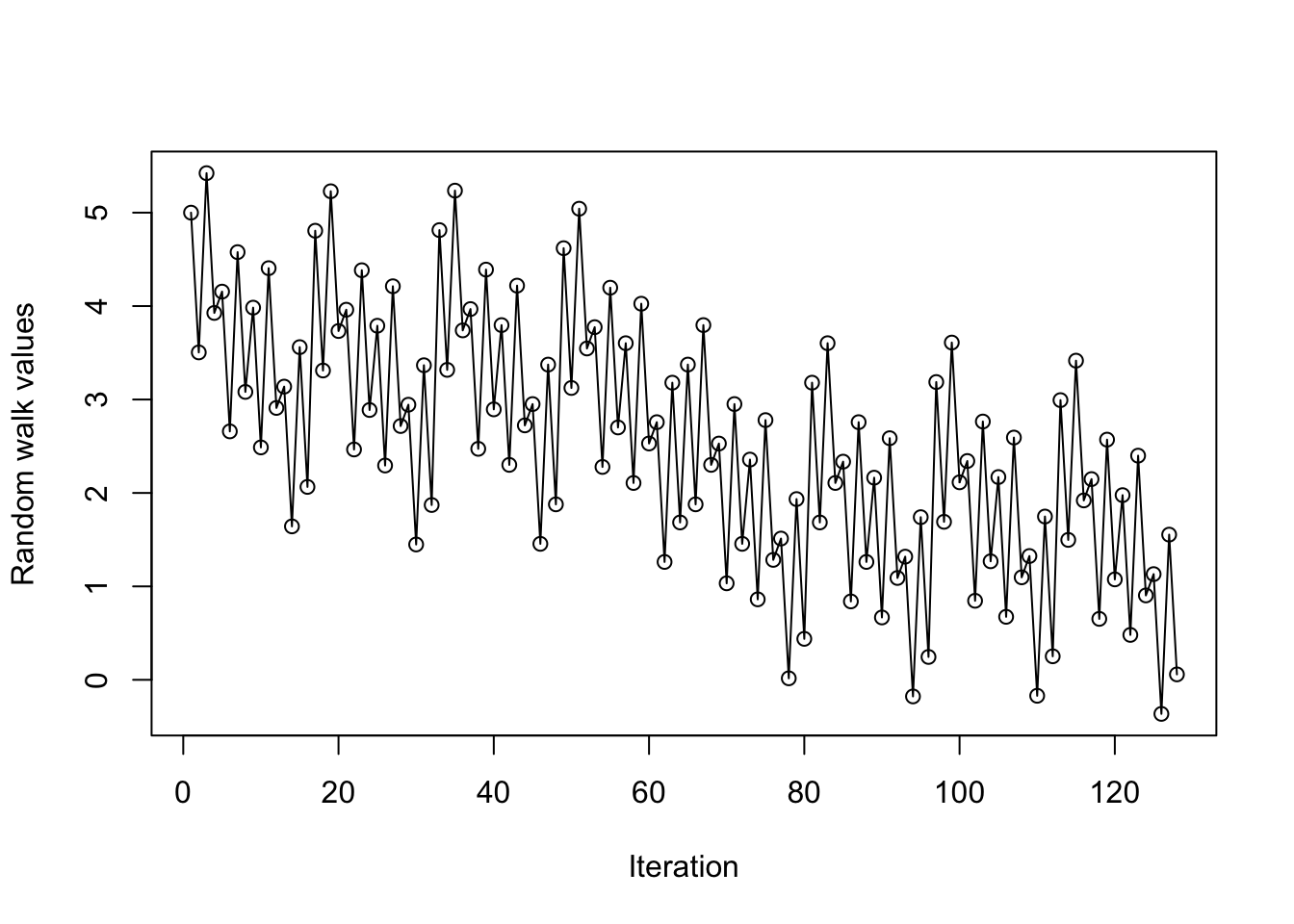Name:
Andrew ID:
Collaborated with:

This lab is to be done in class (completed outside of class time if need be). You can collaborate with your classmates, but you must identify their names above, and you must submit your own lab as an knitted PDF file on Gradescope, by Friday 9pm, this week.

This week’s agenda: practicing debugging with `cat()`, `print()`, and `browser()`; simple testing.

# Q1. Bug hunt practice

In this section of the lab, you will fix a bunch of buggy function definitions. Probably the easiest workflow is to define the function in your console, and then run the sample commands—they will either give errors or produce the wrong outputs. Using any combination of: reading the error messages, `traceback()`, and `cat()` or `print()`, you must find and fix the bugs. Sometimes it can also help to try multiple different inputs, i.e., try new function calls, rather than just looking at the sample calls given to you, in order to determine the bugs. You shouldn’t show any of your debugging work in your final knitted answers—so, don’t show calls to `traceback()`, and don’t leave any `cat()` or `print()` calls in the final, fixed function. (You don’t have to do anything yet, this was just to setup this section of the lab.)

• 1a. Below is a function called `get.cols.with.ab.zeros()`, but it has a few bugs. A few sample matrices are given below in `mat`, `identity.mat`, along with some sample calls that give errors. After fixing the bugs, the calls to `get.cols.with.ab.zeros()` should produce the outputs as described in comments.
``````# Function: cols.with.ab.zeros, to retrieve columns of matrix that have between
#   a and b zeros, each
# Inputs:
# - my.mat: the original matrix
# - a: lower bound for number of zeros allowed; default is 0
# - b: upper bound for number of zeros allowed; default is Inf
# Output: the new matrix

cols.with.ab.zeros = function(my.mat, a=0, b=Inf) {
zeros.per.column = colSums(mat != 0)
i.to.keep = a <= zeros.per.column && zeros.per.column <= b
return(my.mat[i.to.keep,])
}

mat = matrix(c(0,0,1,0,1,1,1,1,1), 3, 3)
identity.mat = diag(1, 3)
cols.with.ab.zeros(mat) # Should get back original matrix``````
``````##      [,1] [,2] [,3]
## [1,]    0    0    1
## [2,]    0    1    1
## [3,]    1    1    1``````
``cols.with.ab.zeros(mat, a=1, b=2) # Should get back first 2 columns of mat``
``````##      [,1] [,2] [,3]
## [1,]    0    0    1
## [2,]    0    1    1
## [3,]    1    1    1``````
``cols.with.ab.zeros(mat, a=2, b=2) # Should get just 1st column of mat; note``
``##      [,1] [,2] [,3]``
``````  # this should still be a matrix though, and not a numeric vector!
cols.with.ab.zeros(identity.mat, a=2, b=2) # Should get back original matrix``````
``##      [,1] [,2] [,3]``
• 1b. Below is a function called `list.extractor()`, but it has a few bugs. A sample list is given below in `cool.list`, along with some sample calls that give errors. After fixing the bugs, the calls to `list.extractor()` should produce the outputs as described in comments.
``````# Function: list.extractor, to extract elements of a list
# Inputs:
# - my.list: the original list
# - i.to.keep: vector of indices, corresponding to elements of the list we
#   want to keep. Default is NULL, in which case this argument is ignored
# - i.to.remove: vector of indices, corresponding to elements of the list we
#   want to remove Default is NULL, in which case this argument is ignored.
#   NOTE: if both i.to.keep and i.to.remove are non-NULL, then the first
#   one should take precedence (i.e., we don't remove anything)
# Output: the new list

list.extractor = function(my.list, i.to.keep=NULL, i.to.remove=NULL) {
if (i.to.keep!=NULL) {
L = my.list[[i.to.keep]]
}
if (i.to.remove!=NULL) {
L = my.list[[-i.to.remove]]
}
return(L)
}

cool.list = list(ints=1:10, lets=letters[1:8], fracs=1:7/7,
bools=sample(c(TRUE,FALSE), 5, replace=TRUE))
list.extractor(cool.list, i.to.keep=c(1,3)) # Should get list with ints, fracs``````
``## Error in if (i.to.keep != NULL) {: argument is of length zero``
``list.extractor(cool.list, i.to.remove=4) # Should get list without bools``
``## Error in if (i.to.keep != NULL) {: argument is of length zero``
``list.extractor(cool.list, i.to.keep=2:4, i.to.remove=4) # Should get list with``
``## Error in if (i.to.keep != NULL) {: argument is of length zero``
``  # lets, fracs, and bools (the i.to.remove argument should be ignored)``
• 1c. Below is a function called `random.walk()`, but it has a few bugs. Some sample calls are given below that produce errors. After fixing the bugs, the calls to `random.walk()` should produce the outputs as described in comment.
``````# Function: random.walk, to run a simple random walk over the reals, which
#   terminates when it reaches 0
# Inputs:
# - x.start: starting position. Default is 5
# - plot.walk: should the result be plotted? Default is TRUE
# - seed: integer seed to pass to set.seed(). Default is NULL, which means
#   effectively no seed is set
# Output: a list with elements x.vals, the values visited by the random walk,
#   and num.steps, the number of steps taken before termination

random.walk = function(x.start=5, plot.walk=TRUE, seed=NULL) {
if (!is.null(seed)) set.seed(seed) # Set the seed, if we need to
x.vals = x.start
while (TRUE) {
r = runif(1, -2, 1)
if (tail(x.vals+r,1) <= 0) break
else x.vals = c(x.vals, x.vals+r)
}
if (plot.walk <- TRUE)
plot(x.vals, xlab="Iteration", ylab="Random walk values", type="o")
return(x.vals=x.vals, num.steps=length(x.vals))
}

random.walk(x.start=5, seed=3)\$num.steps # Should print 8 (this is how many``````
``## Error in return(x.vals = x.vals, num.steps = length(x.vals)): multi-argument returns are not permitted````````  # steps it took the random walk), and produce a plot
random.walk(x.start=10, seed=7)\$num.steps # Should print 14 (this is how many``````
``## Error in return(x.vals = x.vals, num.steps = length(x.vals)): multi-argument returns are not permitted``
``````  # steps it took the random walk), and produce a plot
random.walk(x.start=10, plot.walk=FALSE, seed=7)\$num.steps # Should print 14 ``````
``## Error in return(x.vals = x.vals, num.steps = length(x.vals)): multi-argument returns are not permitted``